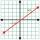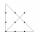Slope

Calculate the slope of a line that intersects points (-84,41) and (-76,-32).

Result

k =  -9.13

Solution:Leave us a comment of this math problem and its solution (i.e. if it is still somewhat unclear...):Be the first to comment!To solve this verbal math problem are needed these knowledge from mathematics:

For Basic calculations in analytic geometry is helpful line slope calculator. From coordinates of two points in the plane it calculate slope, normal and parametric line equation(s), slope, directional angle, direction vector, the length of segment, intersections the coordinate axes etc.

Next similar math problems:

1. V - slopeThe slope of the line whose equation is -3x -9 = 0 is
2. Slope formFind the equation of a line given the point X(8, 1) and slope -2.8. Arrange your answer in the form y = ax + b, where a, b are the constants.
3. PerpendicularWhat is the slope of the perpendicular bisector of line segment AB if A[9,9] and B[9,-2]?
4. Line segmentFor the line segment whose endpoints are L[-1, 13] and M[18, 2], find the x and y value for the point located 4 over 7 the distance from L to M.
5. Line segmentThe 4 cm long line segment is enlarged in the ratio of 5/2. How many centimeters will measure the new line segment?
6. Lie/do not lieThe function is given by the rule f(x) = 8x+16. Determine whether point D[-1; 8] lies on this function. Solve graphically or numerically and give reasons for the your answer.
7. Midpoint of segmentPoint A has coordinates [-16; 23] and the midpoint of the segment AB is the point [2; 12]. What are the coordinates of point B?
8. CandyPeter had a sachet of candy. He wanted to share with his friends. If he gave them 30 candies, he would have 62 candies. If he gave them 40 candies, he would miss 8 candies. How many friends did Peter have?
9. Waiting roomIn the waiting room are people and flies. Together they have 15 heads and 50 legs (fly has 6 legs). How many people and flies are in the waiting room?
10. Arble bagA marble bag sold by Rachel's Marble Company contains 5 orange marbles for every 6 green marbles. If a bag has 35 orange marbles, how many green marbles does it contain?
11. Two numbersFind two numbers whose difference and ratio is 2.
12. DonutsFind how many donuts each student will receive if you share 126 donuts in a ratio of 1:5:8
13. 1.5 divided1.5 divided by 1 = w divided by 4If Petra read 10 pages per day, she would read the book two days earlier than she read 6 pages a day. How many pages does a book have?The enrollment at a local college increased 4% over last year's enrollment of 8548. Find the increase in enrollment (x1) and the current enrollment (x2).Determine the percentage rate of keeper interventions if from 32 shots doesn't caught four shots.How many three-digit odd numbers divisible by 5, which are in place ten's number 3?